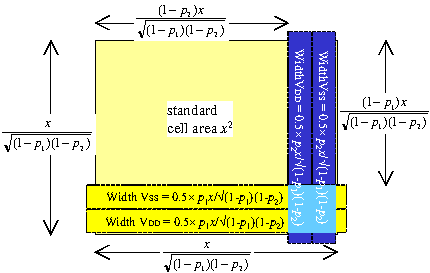If we have a metal power supply using fraction p of the metal resources, and a core side of x, then the increase in the length of the core is not (x×p)%.

 It is x √((1-k(2)×p)×(1-k(3)×p))

referring to the figure on the right.

For example, for a power strap percentage of 15% both horizontally and vertically, this means that the increase in the core side is not 15% but 17.6%.

 Increase = 1 √((1-1×0.15)×(1-1×0.15)) = 1 0.85 = 0.176

We can see this from the drawing on the right. Before power strap insertion, the die area is

 A = x2

and after power strap insertion it is

 A = x2 × (1-k(2)p) × (1-k(3)p)

So that the ratio between the areas after and before power strap insertion is

 (1-k(2)p) × (1-k(3)p)

and the ratio in the length of the side is

 √((1-k(2)p)(1-k(3)p))

In the special case where we make the horizontal and vertical power straps equal, then k(2)=k(3)=1 and a core side of length x will increase to

 x   . 1-pBoth metal-1 and metal-3 horizontal power straps will block the placement of standard cells underneath. This means that one should aim to keep the fraction of metal-1 used for power straps the same as the fraction of metal-3. That is, p1 = p3, and they are used interchangeability in this paper.